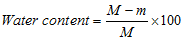water content

(redirected from Residual water content)
Also found in: Encyclopedia.

water content

Water in a contact lens expressed as a percentage of the total mass of the lens in its hydrated state under equilibrium conditions with physiological saline solution containing 9 g/l sodium chloride at a temperature of 20 ± 0.5ºC and with a stated pH value.where M is the mass of hydrated lens, m is the mass of dry lens.The US Food and Drug Administration (FDA) has categorized hydrogel contact lenses into four groups according to their water content and their surface reactivity (referred to as ionic if it contains more than 0.2% ionic material, and nonionic otherwise). Group 1: water content less than 50% and non-ionic. Group 2: water content greater than 50% and non-ionic. Group 3: water content less than 50% and ionic. Group 4: water content greater than 50% and ionic.
References in periodicals archive ?
where [[theta].sub.w] is the volumetric water content; [[theta].sub.s] is the saturated volumetric water content; h is the matric suction; and [a.sub.f], [b.sub.f], [c.sub.f], and [h.sub.r] are fitting coefficients, which are primarily a function of the air entry value, rate of water extraction from the soil, residual water content, and suction at which the residual water content occurs, respectively.
where [theta] represents the volumetric water content function, [[theta].sub.r] represents the residual water content and [[theta].sub.s] represents the saturated water content.
Nano carbon VG content (%) [theta]s [theta]r [alpha] n [R.sup.2] ([cm.sup.3] ([cm.sup.3] ([cm.sup.-1]) [cm.sup.-3]) [cm.sup.-3]) 0 0.4907 0.0878 0.01896 1.772 0.997 0.1 0.5088 0.0990 0.01910 1.668 0.997 0.5 0.5210 0.0917 0.02588 1.539 0.992 0.7 0.5283 0.0940 0.02279 1.527 0.997 1 0.5375 0.0983 0.02779 1.509 0.993 Nano carbon BC content (%) [alpha] n [R.sup.2] ([cm.sup.-1]) 0 0.02792 0.658 0.996 0.1 0.02859 0.573 0.994 0.5 0.03352 0.499 0.985 0.7 0.03219 0.471 0.992 1 0.03539 0.475 0.986 Note: [R.sup.2] is the coefficient of determination for the fit of the VG or BC model to the experimental data; [theta]s, saturated water content; [theta]r, residual water content; [alpha], empirical parameter; n, pore-size distribution parameter Table 5.
Valiantzas  combined Brooks-Corey and Van Genuchten closed-form models improving prediction of conductivity values near the residual water content and saturation.
2008, 2012); C is the residual water content, [A.sub.1] and [A.sub.2] are the water contents of the textural and structural pore spaces at saturation, and [P.sub.1] and [P.sub.2] are applied air pressures characteristic for emptying the textural and structural pore spaces, respectively.
Abbreviations: Se = the effective degree of saturation, th = the volumetric water content, thr = residual water content, ths = saturated volumetric water content, thm, thim= mobile and immobile water content, respectively, thFC = volumetric water content of soil at field capacity (cm3 cm-3); thWP = volumetric water content of soil at wilting point (cm3 cm-3).
Commercially available lubricating oils are delivered by most manufacturers with low residual water content.
Before flooding, measurements of [theta] and h were made in order to estimate residual water content Or from measured low water content under dry conditions for the soil, which is also one of the model parameters.
where [theta]s is the saturated water content ([m.sup.3][m.sup.3]), Or is the residual water content ([m.sup.3][m.sup.-3]), and n is an adjustable parameter that describes the shape of the curve.
where [[theta].sub.s] is the saturated water content ([L.sup.3]/[L.sup.3]), [[theta].sub.r] is the residual water content ([L.sup.3]/[L.sup.3]), 1/[h.sub.e] is a scaling parameter, defined as the inflection point of the curve where [h.sub.e](L) is often described as the air entry parameter, n is a slope parameter, and m is a symmetry parameter with m = 1-[2/n], often referred to as the Burdine restriction (Burdine 1953).
The parameters that were directly calibrated by fitting the model's prediction to observed data were: boundary pressure potential, boundary water content, residual water content, pore size distribution index in micropores, boundary hydraulic conductivity, tortuosity factors in macropores and micropores, dispersivity, and mixing-depth.

Site: Follow: Share:
Open / Close